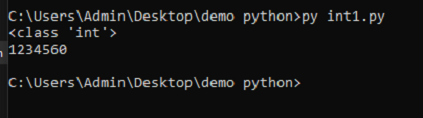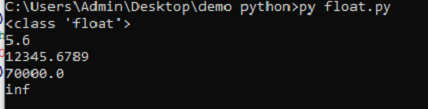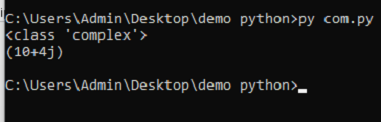# NUMERIC DATA-TYPE

#### NUMERIC DATA-TYPE

As we already know, data types are divided into two main categories boolean and numeric. Numeric is further divided into two parts one is for pure number data types, and another one is for character values. In number data types, we have three distinct data types:

• int
• float
• complex

To check any number data type, we can simply write “type(variavle_name).”

#### Int Data Type

Boolean is a subtype of int data type. Boolean has fixed numbers, but int data type has unlimited precision. It consists of positive and negative values. Python does not define any limit for its int data type.

#### Float Data Type

In C language, the float is implemented by using double. The float data type is more precise than the integer data type, represented by decimal numbers. Float can also be positive and negative values as int have.

#### Complex Data Type

Complex data type does not support many operations like the int and float data types.
E.g., x // y.

#### EXAMPLE CODE

x=7000000000000000000000000000000000000000000000000000000000000000000000000000000 print(type(x)) y=123_456_0 print(y)

#### OUTPUT• FLOAT DATATYPE: Float data type is used to sore decimal or floating-point numbers. Float has the maximum size depending on the type of system you are using. It is denoted by dot(.), E or e.

#### EXAMPLE CODE

f=5.6 #checking the type of f print(type(f)) #printing the float print (f) #printing using delimeter f1=123_45.678_90 print (f1) #printing using e f2=7e4 print(f2) #seeing how size can be in float datatype f3=7e1000 print(f3)

#### OUTPUT• COMPLEX NUMBER: This data type contains an ordered pair of x+jy type where x and y represent the real and imaginary parts respectively. X represents the real part of the number and y represents the imaginary part of the number which is either represented by j or J.

#### EXAMPLE CODE

#creating a complex datatype A=10+4j print(type(A)) print (A)

#### OUTPUT